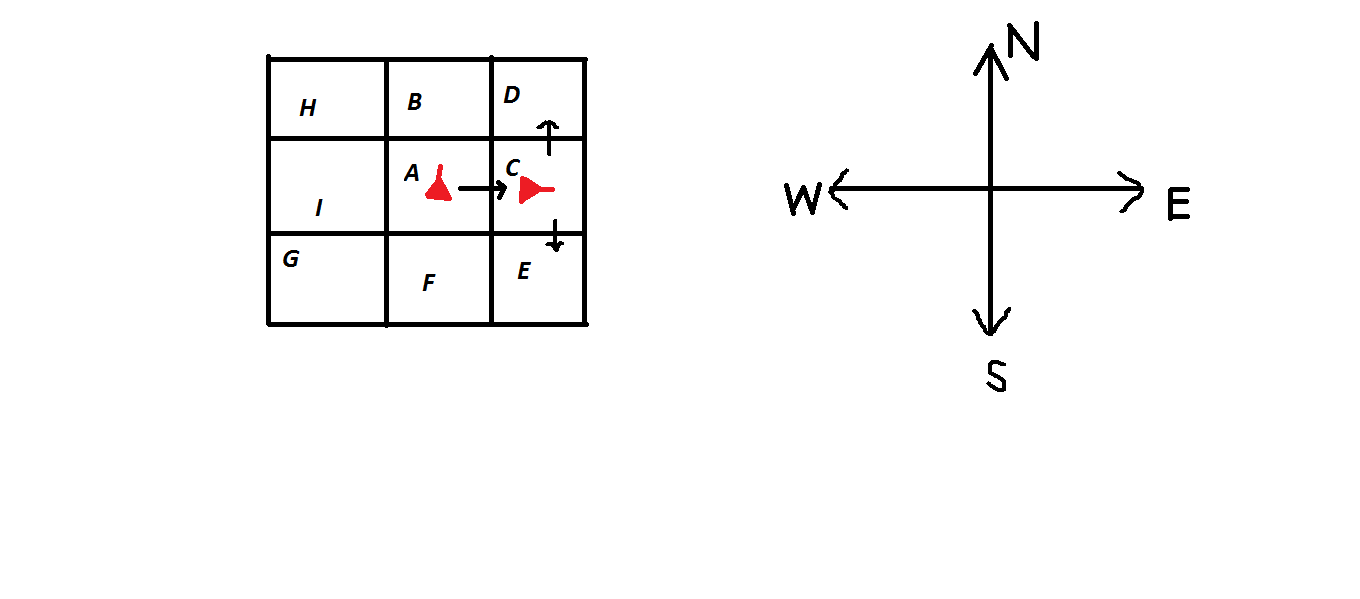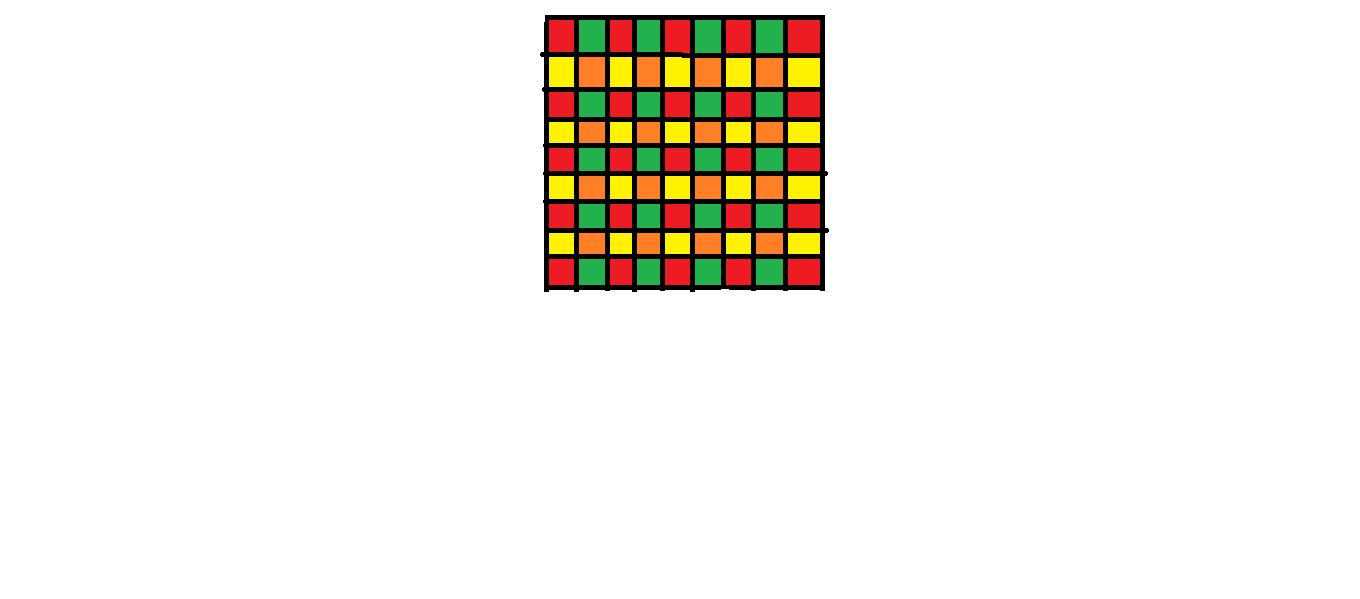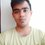# A coloring problem

Coloring problems are, I think, one of the most elegant ways of visualising a problem, that might otherwise be impossible or increasingly difficult to handle.

Here I present a problem, and my coloring solution that really lifted my spirits.

The problem was something like this:

On a $9x9$ grid, two squares are said to be neighboring if they share a common side. There are 65 bugs, each occupying a single square. At t=0, each bug moves to any of the neighboring squares of the square it was previously in. After t=0, each of the bug moves into a square that is to the right of it, or to the left of the square it is presently in. The orientation of the bug, is decided in the following way,Suppose a bug is at the square $A$ at first, and at t=0, it decides to move into square $C$ (It could have moved to $B$ or $I$ or $F$ as well at t=0). The head of the bug is shown by the pointer at its tip. Now it is facing east and it has two options, either to move to $D$ or to $E$, which are to the left and right of it at this moment.

( Note that the initial position of the pointer of the bug is pointed towards $B$ but then it decides to go towards the right, this is because at $A$ it's choices are totally random, so it can actually go to any of the neighboring squares.)

We need to prove that at some point of time t, there will exist a square containing more than one bug.

Solution

First we begin by noting that by the given move scheme, the bug will be on $H$ or $D$ or $E$ or $G$ square after 2 moves, So after 2 moves, the bug will have moved one place along a diagonal in any direction.

According to that scheme here is my coloring:We set the notation by defining that the set of all squares colored red is $R$, that consisting of orange colored squares is $O$, and correspondingly $Y$ and $G$ for those containing yellow and green colored squares.

We note that all the bugs from $R$ will migrate to $O$ after 2 moves and vice versa, and all the bugs from $Y$ will migrate to $G$ after 2 moves and vice versa.

So we have by our colouring |$R$|=25, |$O$|=16, |$Y$|=20, |$G$|=20. Now by Pigeon-Hole Principle we have that there are 65 bugs and 4 kinds of coloured squares they can be put in.

So there are at least $17$ bugs all of which occupy squares of the same color.

In the next two cases we are going to talk about these $17$ bugs exclusively.

Case 1

If they are all initially in $O$, then we are done, because 17 bugs in 16 squares ensures more than one bug in at least one square by the Pigeon Hole Principle.

Case 2

If all of them initially in $R$, then after 2 moves all $17$ will be in $O$, whence we are done again, by the Pigeon Hole Principle as in Case 1.

Another case can happen for which we make the following couple of observations.

After one move, a bug from $R$ or $O$ will always land in $Y$ or $G$.

Case

So, in the third case, we apply Pigeon Hole Principle in the following way:

We have 65 bugs and two classes of colored squares $A$ (consisting of the squares from $R$ and $O$ ) and $B$ (consisting of the squares from $Y$ and $G$ ). So there must be at least 33 bugs in one of the two classes $A$ and $B$.

If the 33 bugs are in class $A$ then there are a total of 33 bugs in $R$ and $O$, so by Pigeon Hole Principle again, there is at least 17 bugs in one of them, and the first 2 cases ensure that more than one bug can be located in the same square in such cases.

If the 33 bugs are in $B$ then after one move they will have moved to $A$, by the observation given above. So after one move we have 33 bugs in A, whence the argument immediately before this ensures more than one bug in one square.

Hence we are done!

Note An interesting thing to note from this proof is that we can achieve the goal of having more than one bug in one square after a maximum of three moves. So at t=3 we can actually ensure that there is more than one bug in some square.

That's the beauty of coloring proofs, a way to visualize.Note by Soumava Pal
5 years, 2 months ago

This discussion board is a place to discuss our Daily Challenges and the math and science related to those challenges. Explanations are more than just a solution — they should explain the steps and thinking strategies that you used to obtain the solution. Comments should further the discussion of math and science.

When posting on Brilliant:

• Use the emojis to react to an explanation, whether you're congratulating a job well done , or just really confused .
• Ask specific questions about the challenge or the steps in somebody's explanation. Well-posed questions can add a lot to the discussion, but posting "I don't understand!" doesn't help anyone.
• Try to contribute something new to the discussion, whether it is an extension, generalization or other idea related to the challenge.
• Stay on topic — we're all here to learn more about math and science, not to hear about your favorite get-rich-quick scheme or current world events.

MarkdownAppears as
*italics* or _italics_ italics
**bold** or __bold__ bold
- bulleted- list
• bulleted
• list
1. numbered2. list
1. numbered
2. list
Note: you must add a full line of space before and after lists for them to show up correctly
paragraph 1paragraph 2

paragraph 1

paragraph 2

[example link](https://brilliant.org)example link
> This is a quote
This is a quote
    # I indented these lines
# 4 spaces, and now they show
# up as a code block.

print "hello world"
# I indented these lines
# 4 spaces, and now they show
# up as a code block.

print "hello world"
MathAppears as
Remember to wrap math in $$ ... $$ or $ ... $ to ensure proper formatting.
2 \times 3 $2 \times 3$
2^{34} $2^{34}$
a_{i-1} $a_{i-1}$
\frac{2}{3} $\frac{2}{3}$
\sqrt{2} $\sqrt{2}$
\sum_{i=1}^3 $\sum_{i=1}^3$
\sin \theta $\sin \theta$
\boxed{123} $\boxed{123}$

## Comments

Sort by:

Top Newest

"After one move, a bug from $R$ will always land in $Y$ and vice versa. Similarly a bug from $O$ will always land in $G$ and vice versa."
I believe you meant,
"After one move, a bug from $R$ or $O$ will always land in $Y$ or $G$ and vice versa."

Otherwise, it's clear, and simple to understand. Thanks for sharing!
Would you mind contributing to the Coloring wiki?

- 5 years, 2 months ago

Log in to reply

@Ameya Daigavane I am so sorry, I totally missed that. I am editing it right now.

Yea I would love to contribute to it.

- 5 years, 2 months ago

Log in to reply

wow

- 5 years, 2 months ago

Log in to reply

×

Problem Loading...

Note Loading...

Set Loading...